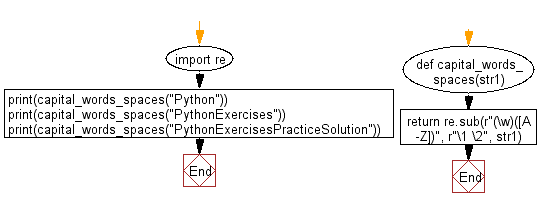﻿ Python Exercises: Insert spaces between words starting with capital letters - w3resource# Python: Insert spaces between words starting with capital letters

## Python Regular Expression: Exercise-51 with Solution

Write a Python program to insert spaces between words starting with capital letters.

Sample Solution:-

Python Code:

``````import re
def capital_words_spaces(str1):
return re.sub(r"(\w)([A-Z])", r"\1 \2", str1)

print(capital_words_spaces("Python"))
print(capital_words_spaces("PythonExercises"))
print(capital_words_spaces("PythonExercisesPracticeSolution"))
```
```

Sample Output:

```Python
Python Exercises
Python Exercises Practice Solution
```

Flowchart:## Visualize Python code execution:

The following tool visualize what the computer is doing step-by-step as it executes the said program:

Python Code Editor:

Have another way to solve this solution? Contribute your code (and comments) through Disqus.

What is the difficulty level of this exercise?

﻿